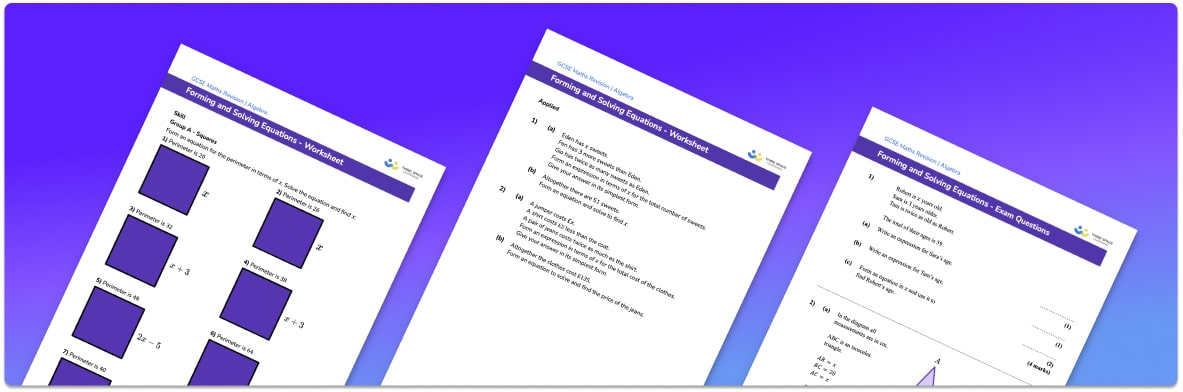# Forming And Solving Equations Worksheet• Section 1 of the forming and solving equations worksheet contains 24 skills-based forming and solving equations practice questions, in 3 groups to support differentiation
• Section 2 contains 5 applied forming and solving equations questions with a mix of worded problems and deeper problem solving questions
• Section 3 contains 4 foundation and higher level GCSE exam style questions on forming and solving equations
• Answers and a mark scheme for all forming and solving equations questions are provided
• Questions follow variation theory with plenty of opportunities for students to work independently at their own level
• All questions created by fully qualified expert secondary maths teachers
• Suitable for GCSE maths revision for AQA, OCR and Edexcel exam boards

• This field is for validation purposes and should be left unchanged.

You can unsubscribe at any time (each email we send will contain an easy way to unsubscribe). To find out more about how we use your data, see our privacy policy.

### Forming and solving equations at a glance

Forming & solving equations involves taking information from a practical scenario and using it to create an equation which can then be solved. The scenario may involve the perimeter or area of a shape or the angles within a shape. For example, we might be given the perimeter of an isosceles triangle and told the lengths of its sides in terms of x or we might be given the size of two angles within a set of parallel lines in terms of y. For both of these scenarios, we could write down an equation which we could then solve to find the values of the unknowns.

An equation could also be written from the information given by a function machine or a word problem. This could be something like: I have a length and I subtract 10 centimetres from the length, I then half the length, leaving me with 40 centimetres. Find the original length.

When forming & solving equations, it is important that we are first confident at solving one step and two step equations, expanding brackets and solving equations with unknowns on both sides. We also need to be confident at applying our knowledge of calculating the area of various shapes including triangles, quadrilaterals, trapeziums and parallelograms, as well as finding the perimeter of polygons and applying angle facts.

Looking forward, students can progress with more solving equations worksheets and to additional algebra worksheets, for example a simplifying expressions worksheetFor more teaching and learning support on Algebra our GCSE maths lessons provide step by step support for all GCSE maths concepts.

## Do you have KS4 students who need more focused attention to succeed at GCSE?There will be students in your class who require individual attention to help them succeed in their maths GCSEs. In a class of 30, it’s not always easy to provide.

Help your students feel confident with exam-style questions and the strategies they’ll need to answer them correctly with our dedicated GCSE maths revision programme.

Lessons are selected to provide support where each student needs it most, and specially-trained GCSE maths tutors adapt the pitch and pace of each lesson. This ensures a personalised revision programme that raises grades and boosts confidence.

Find out more